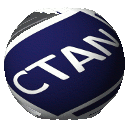# bodeplot

package to plot Bode, Nichols, and Nyquist diagrams.

Inspired by the `bodegraph` package.

Version 1.0.8 and newer store `gnuplot` temporary files in the working directory. Use class option `declutter` to restore pre-v1.0.8 behavior. Option `declutter` can cause errors if used with a `tikzexternalize` prefix.

Compilation instructions: 1) `latex bodeplot.ins` to generate `bodeplot.sty` 2) To compile documentation (needs `gnuplot` on system PATH):

```pdflatex bodeplot.dtx --shell-escape
makeindex -s gind.ist bodeplot.idx
makeindex -s gglo.ist -o bodeplot.gls bodeplot.glo
pdflatex bodeplot.dtx --shell-escape
pdflatex bodeplot.dtx --shell-escape```

• New `\BodeZPK` and `\BodeTF` commands to generate Bode plots of any transfer function given either poles, zeros, gain, and delay, or numerator and denominator coefficients and delay
• Support for unstable poles and zeros.
• Support for complex poles and zeros.
• Support for general stable and unstable second order transfer functions.
• Support for both `gnuplot` (default) and `pgfplots` (package option `pgf`).
• Support for linear and asymptotic approximation of magnitude and phase plots of any transfer function given poles, zeros, and gain.

Main Bode/Nyquist/Nichols commands: Given Zeros, Poles, Gain, and Delay (Bode plots support asymptotic and linear approximation for systems without delays):

• `\BodeZPK[object1/type1/{options1},object2/type2/{options2},...]{z/{zeros},p/{poles},k/gain,d/delay}{min-frequency}{max-frequency}`
• `\NicholsZPK[plot/{options},axes/{options}]{z/{zeros},p/{poles},k/gain,d/delay}{min-frequency}{max-frequency}`
• `\NyquistZPK[plot/{options},axes/{options}]{z/{zeros},p/{poles},k/gain,d/delay}{min-frequency}{max-frequency}`

Given Numerator and denominator coefficients and delay (does not support approximation yet):

• `\BodeTF[object1/type1/{options1},object2/type2/{options2},...]{num/{coeff},den/{coeff},d/delay}{min-frequency}{max-frequency}`
• `\NicholsTF[plot/{options},axes/{options}]{num/{coeff},den/{coeff},d/delay}`
• `\NyquistTF[plot/{options},axes/{options}]{num/{coeff},den/{coeff},d/delay}`

Other new environments and associated commands:

• `BodePlot` environment
• `\addBodeZPKPlots[{approximation1/{plot-options1}},{approximation2/{plot-options2}},...]{plot-type (phase or magnitude)}{z/{zeros},p/{poles},k/gain,d/delay}`
• `\addBodeTFPlot[plot-options]{plot-type (phase or magnitude)}{num/{coeff},den/{coeff},d/delay}`
• `\addBodeComponentPlot[plot-options]{basic_component_plot_command}`
• Basic component plot commands: (append `Lin` to get linear approximation and `Asymp` to get asymptotic approximation) (change `Pole` to `Zero` to get inverse plots) (change `Mag` to `Ph` to get phase plots)
• `\MagK{a}` - Pure gain, G(s) = a.
• `\MagPole{a}{b}` - Single pole at s = a+bi, G(s) = 1/(s - a-bi).
• `\MagCSPoles{z}{w}` - Cannonical Second order system, G(s) = 1/(s^2 + 2zws + w^2).
• `\MagSOPoles{a}{b}` - Second Order system, G(s) = 1/(s^2 + as + b).
• `\MagDel{T}` - Pure delay, G(s) = exp(-Ts) (does not admit asymptotic approximation).
• `NicholsChart` environment
• `\addNicholsZPKChart[plot-options]{z/{zeros},p/{poles},k/gain,d/delay}`
• `\addNicholsTFChart[plot-options]{num/{coeff},den/{coeff},d/delay}`
• `NyquistPlot` environment
• `\addNyquistZPKPlot[plot-options]{z/{zeros},p/{poles},k/gain,d/delay}`
• `\addNyquistTFPlot[plot-options]{num/{coeff},den/{coeff},d/delay}`

Limitation: TF commands are wrapped between 0 and 360 degrees in `pgf` mode.

## bodeplot – Draw Bode, Nyquist and Nichols plots with gnuplot or pgfplots

This is a package to plot Bode, Nichols, and Nyquist diagrams. It provides added functionality over the similar bodegraph package:

• New \BodeZPK and \BodeTF commands to generate Bode plots of any transfer function given either poles, zeros, gain, and delay, or numerator and denominator coefficients and delay
• Support for unstable poles and zeros.
• Support for complex poles and zeros.
• Support for general stable and unstable second order transfer functions.
• Support for both Gnuplot (default) and pgfplots (package option pgf).
• Support for linear and asymptotic approximation of magnitude and phase plots of any transfer function given poles, zeros, and gain.

 Package bodeplot Bug tracker https://github.com/rlkamalapurkar/bodeplot/issues Repository https://github.com/rlkamalapurkar/bodeplot Version 1.1.1 2022-07-31 Licenses The LaTeX Project Public License 1.3c Copyright 2021 Rushikesh Kamalapurkar Maintainer Rushikesh Kamalapurkar Contained in TeX Live as bodeplotMiKTeX as bodeplot Topics Graphics plotPGF TikZEngineering See also bodegraph